Electron. J. Diff. Equ., Vol. 2009(2009), No. 131, pp. 1-16.

### Multiple solutions for nonlinear elliptic equations on Riemannian manifolds Wenjing Chen, Jianfu Yang

Abstract:
Let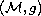be a compact, connected, orientable, Riemannian-manifold of class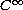with Riemannian metric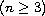. We study the existence of solutions to the equation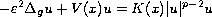on this Riemannian manifold. Here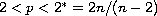,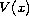and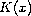are continuous functions. We show that the shape ofandaffects the number of solutions, and then prove the existence of multiple solutions.

Submitted September 15, 2009. Published October 9, 2009.
Math Subject Classifications: 35J20, 35J61, 58J05.
Key Words: Multiple Solutions; Semilinear elliptic equation; Riemannian manifold; Ljusternik-Schnirelmann category.

Show me the PDF file (302 KB), TEX file, and other files for this article.

 Wenjing Chen Department of Mathematics, Jiangxi Normal University Nanchang, Jiangxi, 330022, China email: wjchen1102@yahoo.com.cn Jianfu Yang Department of Mathematics, Jiangxi Normal University Nanchang, Jiangxi, 330022, China email: jfyang_2000@yahoo.com